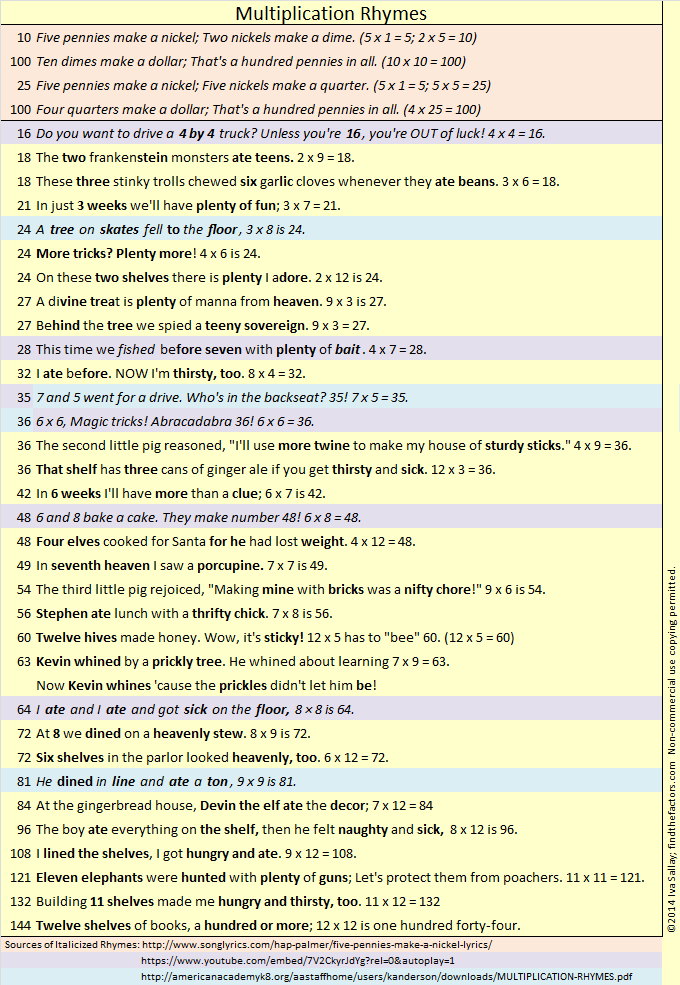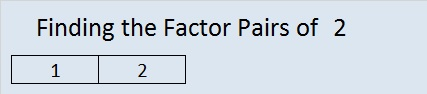# 2 Gross Multiplication FactsThis level TWO multiplication table is only a little more difficult than the table in my previous post.  This puzzle uses 12 factors while the previous one used just 10. To find all these factors you have to be familiar with 144 multiplication facts, a requirement that sounds much more intimidating than it really is. (For example, 3 x 5 and 5 x 3 are counted as 2 different facts.) 144 is a fascinating number.  It is a dozen dozen, otherwise known as a gross. Therefore, completing this puzzle will help you review the gross multiplication facts!   If you haven’t memorized all of the facts, there is a gross way to learn some of the gross multiplication facts.

Edwin A. Anderson Elementary School uses manipulatives and rhymes to teach the multiplication facts.  Their students learn two rhymes a week for sixteen week and are masters of the gross multiplication facts when they are done. Five of the rhymes are actually a little gross:

• 3×3, nine cuts on my knee
• 3×8, 24 horseflies on my plate
• 4×7, 28 spiders are webbin’
• 6×8, fishing bait, count slimy worms all 48
• 7×7, roaches on a vine, yucky and scary all 49

It was a very gross multiplication rhyme about the number 64 that inspired me to collect some favorite rhymes and even write a few rhymes myself. I collected them in one list I titled Multiplication Rhymes:That gross, but inspiring, rhyme about the number 64 and some other gross multiplication rhymes are listed below:

• 18 The two Frankenstein monsters ate teens. 2×9=18
• 18 These three stinky trolls chewed six garlic cloves whenever they ate beans. 3×6=18
• 36 That shelf has three cans of ginger ale if you get thirsty and sick. 12×3=36
• 63 Kevin whined by a prickly tree. He whined about learning 7×9=63. Now Kevin whines ’cause the prickles didn’t let him be!
• 64 I ate and I ate and got sick on the floor, 8×8 is 64.
• 96 The boy ate everything on the shelf. Then he felt naughty and sick. 8×12 is 96.

Knowing all 144 (gross) multiplication facts will help you complete my puzzles.

As you Find the Factors and fill in those gross multiplication facts, there are two things I’d like you to remember:

1. Don’t write anything on the inside of the puzzle until you write down all the factors on the outside of the puzzle.

2. The only numbers allowed in the factor row (top row) and the factor column (1st column) are the numbers from 1 to 12, and all 12 numbers must appear in both places.

Now you can click on 12 Factors 2013-10-31, to find the 6 puzzles appearing in this post.

Now let’s think about the number 2:

2 is the smallest prime number and the only prime that is an even number.

2 is also the largest number that is equal to its number of factors.

In particular situations, there are several prefixes and words that mean two:

• 2 is the second counting number.
• A bicycle is a two-wheeler. A binomial is a polynomial with two terms.
• Two musicians form a duet. Take a look at duet’s synonyms and translations in other languages.
• The playing card with a 2 on it is called the deuce.
• A pair can be two shoes, two eyes, or lots of other possibilities.
• Two people in a relationship are called a couple.

Can you think of any more?

Base 2 uses only the digits 1 and 0 to represent each counting number. Computers use base 2, or binary, as it is also called.

Wikipedia informs us of a Calculus topic about the number 2:

• If you take the sum from 0 to ∞ of b⁻ᵏ, it will converge to b, ONLY when the base number, b, is 2. Thus,
•  ⅟₁ + ⅟₂ + ⅟₄ + ⅟₈ + ⅟₁₆ + ⅟₃₂ + ⅟₆₄ + ⅟₁₂₈ + … = 2

Here is 2’s factoring information:

• 2 is a prime number.
• Prime factorization: 2 is prime.
• The exponent of prime number 2 is 1. Adding 1 to that exponent we get (1 + 1) = 2. Therefore 2 has exactly 2 factors.
• Factors of 2: 1, 2
• Factor pairs: 2 = 1 x 2
• 2 has no square factors that allow its square root to be simplified. √2 ≈ 1.414.How do we know that 2 is a prime number? If 2 were not a prime number, then it would be divisible by at least one prime number less than or equal to √2 ≈ 1.4. Since there are no prime numbers less than or equal to 1.4, we know 2 is a prime number.

When 2 is a clue in the FIND THE FACTOR puzzles, the factors are always 1 and 2.

This site uses Akismet to reduce spam. Learn how your comment data is processed.